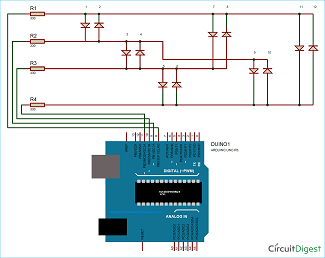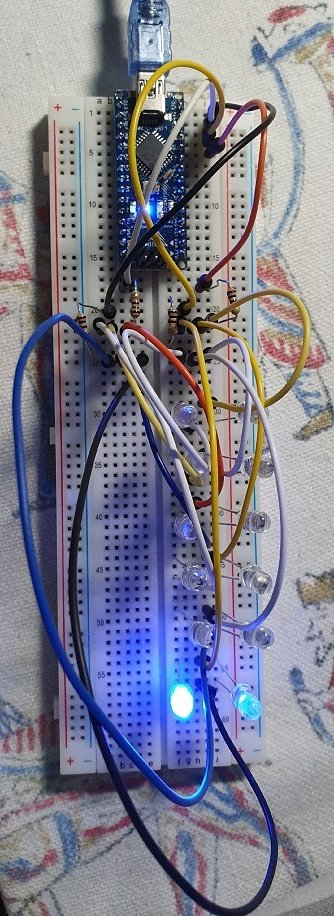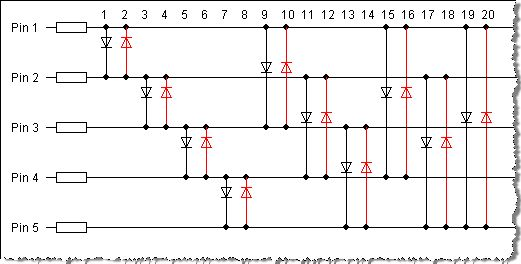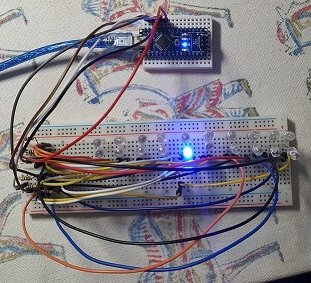# B4R TutorialCharlieplexing - controlling N*(N-1) LED's with N GPIO pins

It is based on this posting. I am using an Arduino Nano to control the 12 LEDs using pins 8, 9, 10, and 11 of the Nano. Just thought it would be interesting to give Charlieplexing a go. Working very nicely...B4X:
``````#Region Project Attributes
#AutoFlushLogs: True
#CheckArrayBounds: True
#StackBufferSize: 300
#End Region

Sub Process_Globals
'These global variables will be declared once when the application starts.
'Public variables can be accessed from all modules.
Public Serial1 As Serial

Dim p8, p9, p10, p11 As Pin

Dim t As Timer
Dim cnt As Int

End Sub

Private Sub AppStart
Serial1.Initialize(115200)
Log("AppStart")

cnt = 0

p8.Initialize(8, p8.MODE_OUTPUT)
p9.Initialize(9, p9.MODE_OUTPUT)
p10.Initialize(10, p10.MODE_OUTPUT)
p11.Initialize(11, p11.MODE_OUTPUT)
t.Initialize("t_tick", 50)

t.Enabled = True
End Sub

Sub t_tick

cnt = cnt + 1
If cnt = 13 Then cnt = 1
If cnt = 1 Then
p8.Initialize(8, p8.MODE_OUTPUT)
p9.Initialize(9, p9.MODE_OUTPUT)
p10.Initialize(10, p10.MODE_INPUT)
p11.Initialize(11, p11.MODE_INPUT)
p8.DigitalWrite(True)
p9.DigitalWrite(False)
End If

If cnt = 2 Then
p8.Initialize(8, p8.MODE_OUTPUT)
p9.Initialize(9, p9.MODE_OUTPUT)
p10.Initialize(10, p10.MODE_INPUT)
p11.Initialize(11, p11.MODE_INPUT)
p8.DigitalWrite(False)
p9.DigitalWrite(True)
End If

If cnt = 3 Then
p8.Initialize(8, p8.MODE_INPUT)
p9.Initialize(9, p9.MODE_OUTPUT)
p10.Initialize(10, p10.MODE_OUTPUT)
p11.Initialize(11, p11.MODE_INPUT)
p9.DigitalWrite(True)
p10.DigitalWrite(False)
End If

If cnt = 4 Then
p8.Initialize(8, p8.MODE_INPUT)
p9.Initialize(9, p9.MODE_OUTPUT)
p10.Initialize(10, p10.MODE_OUTPUT)
p11.Initialize(11, p11.MODE_INPUT)
p9.DigitalWrite(False)
p10.DigitalWrite(True)
End If

If cnt = 5 Then
p8.Initialize(8, p8.MODE_INPUT)
p9.Initialize(9, p9.MODE_INPUT)
p10.Initialize(10, p10.MODE_OUTPUT)
p11.Initialize(11, p11.MODE_OUTPUT)
p10.DigitalWrite(True)
p11.DigitalWrite(False)
End If

If cnt = 6 Then
p8.Initialize(8, p8.MODE_INPUT)
p9.Initialize(9, p9.MODE_INPUT)
p10.Initialize(10, p10.MODE_OUTPUT)
p11.Initialize(11, p11.MODE_OUTPUT)
p10.DigitalWrite(False)
p11.DigitalWrite(True)
End If

If cnt = 7 Then
p8.Initialize(8, p8.MODE_OUTPUT)
p9.Initialize(9, p9.MODE_INPUT)
p10.Initialize(10, p10.MODE_OUTPUT)
p11.Initialize(11, p11.MODE_INPUT)
p8.DigitalWrite(True)
p10.DigitalWrite(False)
End If

If cnt = 8 Then
p8.Initialize(8, p8.MODE_OUTPUT)
p9.Initialize(9, p9.MODE_INPUT)
p10.Initialize(10, p10.MODE_OUTPUT)
p11.Initialize(11, p11.MODE_INPUT)
p8.DigitalWrite(False)
p10.DigitalWrite(True)
End If

If cnt = 9 Then
p8.Initialize(8, p8.MODE_INPUT)
p9.Initialize(9, p9.MODE_OUTPUT)
p10.Initialize(10, p10.MODE_INPUT)
p11.Initialize(11, p11.MODE_OUTPUT)
p9.DigitalWrite(True)
p11.DigitalWrite(False)
End If

If cnt = 10 Then
p8.Initialize(8, p8.MODE_INPUT)
p9.Initialize(9, p9.MODE_OUTPUT)
p10.Initialize(10, p10.MODE_INPUT)
p11.Initialize(11, p11.MODE_OUTPUT)
p9.DigitalWrite(False)
p11.DigitalWrite(True)
End If

If cnt = 11 Then
p8.Initialize(8, p8.MODE_OUTPUT)
p9.Initialize(9, p9.MODE_INPUT)
p10.Initialize(10, p10.MODE_INPUT)
p11.Initialize(11, p11.MODE_OUTPUT)
p8.DigitalWrite(True)
p11.DigitalWrite(False)
End If

If cnt = 12 Then
p8.Initialize(8, p8.MODE_OUTPUT)
p9.Initialize(9, p9.MODE_INPUT)
p10.Initialize(10, p10.MODE_INPUT)
p11.Initialize(11, p11.MODE_OUTPUT)
p8.DigitalWrite(False)
p11.DigitalWrite(True)
End If

End Sub``````

#### Attachments

• b4rCharliePlexing.zip
1.1 KB · Views: 217

#### Johan Schoeman

##### Expert
Longtime User
Based on this tutorial - controlling 20 LEDs with 5 GPIO pins on an Arduino (in this case using a Nano)....A bit of a crow's nest with the wiring - but working perfectly...B4X:
``````#Region Project Attributes
#AutoFlushLogs: True
#CheckArrayBounds: True
#StackBufferSize: 300
#End Region

Sub Process_Globals
'These global variables will be declared once when the application starts.
'Public variables can be accessed from all modules.
Public Serial1 As Serial

Dim p7, p8, p9, p10, p11 As Pin

Dim delaytime As ULong = 100

End Sub

Private Sub AppStart
Serial1.Initialize(115200)
Log("AppStart")

p7.Initialize(7, p7.MODE_OUTPUT)
p8.Initialize(8, p8.MODE_OUTPUT)
p9.Initialize(9, p9.MODE_OUTPUT)
p10.Initialize(10, p10.MODE_OUTPUT)
p11.Initialize(11, p11.MODE_OUTPUT)

Delay(1000)

light_1(0)

End Sub

Sub light_1(tag As Byte)

p7.Initialize(7, p7.MODE_OUTPUT)
p8.Initialize(8, p8.MODE_OUTPUT)
p9.Initialize(9, p9.MODE_INPUT)
p10.Initialize(10, p10.MODE_INPUT)
p11.Initialize(11, p11.MODE_INPUT)
p7.DigitalWrite(True)
p8.DigitalWrite(False)
CallSubPlus("light_3", delaytime, 0)
End Sub

Sub light_2(tag As Byte)

p7.Initialize(7, p7.MODE_OUTPUT)
p8.Initialize(8, p8.MODE_OUTPUT)
p9.Initialize(9, p9.MODE_INPUT)
p10.Initialize(10, p10.MODE_INPUT)
p11.Initialize(11, p11.MODE_INPUT)
p7.DigitalWrite(False)
p8.DigitalWrite(True)
CallSubPlus("light_1", delaytime, 0)
End Sub

Sub light_3(tag As Byte)

p7.Initialize(7, p7.MODE_INPUT)
p8.Initialize(8, p8.MODE_OUTPUT)
p9.Initialize(9, p9.MODE_OUTPUT)
p10.Initialize(10, p10.MODE_INPUT)
p11.Initialize(11, p11.MODE_INPUT)
p8.DigitalWrite(True)
p9.DigitalWrite(False)
CallSubPlus("light_5", delaytime, 0)
End Sub

Sub light_4(tag As Byte)

p7.Initialize(7, p7.MODE_INPUT)
p8.Initialize(8, p8.MODE_OUTPUT)
p9.Initialize(9, p9.MODE_OUTPUT)
p10.Initialize(10, p10.MODE_INPUT)
p11.Initialize(11, p11.MODE_INPUT)
p8.DigitalWrite(False)
p9.DigitalWrite(True)
CallSubPlus("light_2", delaytime, 0)
End Sub

Sub light_5(tag As Byte)

p7.Initialize(7, p7.MODE_INPUT)
p8.Initialize(8, p8.MODE_INPUT)
p9.Initialize(9, p9.MODE_OUTPUT)
p10.Initialize(10, p10.MODE_OUTPUT)
p11.Initialize(11, p11.MODE_INPUT)
p9.DigitalWrite(True)
p10.DigitalWrite(False)
CallSubPlus("light_7", delaytime, 0)
End Sub

Sub light_6(tag As Byte)

p7.Initialize(7, p7.MODE_INPUT)
p8.Initialize(8, p8.MODE_INPUT)
p9.Initialize(9, p9.MODE_OUTPUT)
p10.Initialize(10, p10.MODE_OUTPUT)
p11.Initialize(11, p11.MODE_INPUT)
p9.DigitalWrite(False)
p10.DigitalWrite(True)
CallSubPlus("light_4", delaytime, 0)
End Sub

Sub light_7(tag As Byte)

p7.Initialize(7, p7.MODE_INPUT)
p8.Initialize(8, p8.MODE_INPUT)
p9.Initialize(9, p9.MODE_INPUT)
p10.Initialize(10, p10.MODE_OUTPUT)
p11.Initialize(11, p11.MODE_OUTPUT)
p10.DigitalWrite(True)
p11.DigitalWrite(False)
CallSubPlus("light_9", delaytime, 0)
End Sub

Sub light_8(tag As Byte)

p7.Initialize(7, p7.MODE_INPUT)
p8.Initialize(8, p8.MODE_INPUT)
p9.Initialize(9, p9.MODE_INPUT)
p10.Initialize(10, p10.MODE_OUTPUT)
p11.Initialize(11, p11.MODE_OUTPUT)
p10.DigitalWrite(False)
p11.DigitalWrite(True)
CallSubPlus("light_6", delaytime, 0)
End Sub

Sub light_9(tag As Byte)

p7.Initialize(7, p7.MODE_OUTPUT)
p8.Initialize(8, p8.MODE_INPUT)
p9.Initialize(9, p9.MODE_OUTPUT)
p10.Initialize(10, p10.MODE_INPUT)
p11.Initialize(11, p11.MODE_INPUT)
p7.DigitalWrite(True)
p9.DigitalWrite(False)
CallSubPlus("light_11", delaytime, 0)
End Sub

Sub light_10(tag As Byte)

p7.Initialize(7, p7.MODE_OUTPUT)
p8.Initialize(8, p8.MODE_INPUT)
p9.Initialize(9, p9.MODE_OUTPUT)
p10.Initialize(10, p10.MODE_INPUT)
p11.Initialize(11, p11.MODE_INPUT)
p7.DigitalWrite(False)
p9.DigitalWrite(True)
CallSubPlus("light_8", delaytime, 0)
End Sub

Sub light_11(tag As Byte)

p7.Initialize(7, p7.MODE_INPUT)
p8.Initialize(8, p8.MODE_OUTPUT)
p9.Initialize(9, p9.MODE_INPUT)
p10.Initialize(10, p10.MODE_OUTPUT)
p11.Initialize(11, p11.MODE_INPUT)
p8.DigitalWrite(True)
p10.DigitalWrite(False)
CallSubPlus("light_13", delaytime, 0)
End Sub

Sub light_12(tag As Byte)

p7.Initialize(7, p7.MODE_INPUT)
p8.Initialize(8, p8.MODE_OUTPUT)
p9.Initialize(9, p9.MODE_INPUT)
p10.Initialize(10, p10.MODE_OUTPUT)
p11.Initialize(11, p11.MODE_INPUT)
p8.DigitalWrite(False)
p10.DigitalWrite(True)
CallSubPlus("light_10", delaytime, 0)
End Sub

Sub light_13(tag As Byte)

p7.Initialize(7, p7.MODE_INPUT)
p8.Initialize(8, p8.MODE_INPUT)
p9.Initialize(9, p9.MODE_OUTPUT)
p10.Initialize(10, p10.MODE_INPUT)
p11.Initialize(11, p11.MODE_OUTPUT)
p9.DigitalWrite(True)
p11.DigitalWrite(False)
CallSubPlus("light_15", delaytime, 0)
End Sub

Sub light_14(tag As Byte)

p7.Initialize(7, p7.MODE_INPUT)
p8.Initialize(8, p8.MODE_INPUT)
p9.Initialize(9, p9.MODE_OUTPUT)
p10.Initialize(10, p10.MODE_INPUT)
p11.Initialize(11, p11.MODE_OUTPUT)
p9.DigitalWrite(False)
p11.DigitalWrite(True)
CallSubPlus("light_12", delaytime, 0)
End Sub

Sub light_15(tag As Byte)

p7.Initialize(7, p7.MODE_OUTPUT)
p8.Initialize(8, p8.MODE_INPUT)
p9.Initialize(9, p9.MODE_INPUT)
p10.Initialize(10, p10.MODE_OUTPUT)
p11.Initialize(11, p11.MODE_INPUT)
p7.DigitalWrite(True)
p10.DigitalWrite(False)
CallSubPlus("light_17", delaytime, 0)
End Sub

Sub light_16(tag As Byte)

p7.Initialize(7, p7.MODE_OUTPUT)
p8.Initialize(8, p8.MODE_INPUT)
p9.Initialize(9, p9.MODE_INPUT)
p10.Initialize(10, p10.MODE_OUTPUT)
p11.Initialize(11, p11.MODE_INPUT)
p7.DigitalWrite(False)
p10.DigitalWrite(True)
CallSubPlus("light_14", delaytime, 0)
End Sub

Sub light_17(tag As Byte)

p7.Initialize(7, p7.MODE_INPUT)
p8.Initialize(8, p8.MODE_OUTPUT)
p9.Initialize(9, p9.MODE_INPUT)
p10.Initialize(10, p10.MODE_INPUT)
p11.Initialize(11, p11.MODE_OUTPUT)
p8.DigitalWrite(True)
p11.DigitalWrite(False)
CallSubPlus("light_19", delaytime, 0)
End Sub

Sub light_18(tag As Byte)

p7.Initialize(7, p7.MODE_INPUT)
p8.Initialize(8, p8.MODE_OUTPUT)
p9.Initialize(9, p9.MODE_INPUT)
p10.Initialize(10, p10.MODE_INPUT)
p11.Initialize(11, p11.MODE_OUTPUT)
p8.DigitalWrite(False)
p11.DigitalWrite(True)
CallSubPlus("light_16", delaytime, 0)
End Sub

Sub light_19(tag As Byte)

p7.Initialize(7, p7.MODE_OUTPUT)
p8.Initialize(8, p8.MODE_INPUT)
p9.Initialize(9, p9.MODE_INPUT)
p10.Initialize(10, p10.MODE_INPUT)
p11.Initialize(11, p11.MODE_OUTPUT)
p7.DigitalWrite(True)
p11.DigitalWrite(False)
CallSubPlus("light_20", delaytime, 0)
End Sub

Sub light_20(tag As Byte)

p7.Initialize(7, p7.MODE_OUTPUT)
p8.Initialize(8, p8.MODE_INPUT)
p9.Initialize(9, p9.MODE_INPUT)
p10.Initialize(10, p10.MODE_INPUT)
p11.Initialize(11, p11.MODE_OUTPUT)
p7.DigitalWrite(False)
p11.DigitalWrite(True)
CallSubPlus("light_18", delaytime, 0)
End Sub``````

#### Attachments

• b4rCharliePlexing20.zip
1.3 KB · Views: 195

#### Johan Schoeman

##### Expert
Longtime User
The example in post #2 works better with the below code as it switches all pin modes back to MODE_INPUT before setting the modes for the pins that need to be MODE_OUTPUT. It eliminates the unwanted flickering of some LED's that might maybe occur when they are supposed to be off.

B4X:
``````#Region Project Attributes
#AutoFlushLogs: True
#CheckArrayBounds: True
#StackBufferSize: 300
#End Region

Sub Process_Globals
'These global variables will be declared once when the application starts.
'Public variables can be accessed from all modules.
Public Serial1 As Serial

Dim p7, p8, p9, p10, p11 As Pin

Dim delaytime As ULong = 50

End Sub

Private Sub AppStart
Serial1.Initialize(115200)
Log("AppStart")

p7.Initialize(7, p7.MODE_INPUT)
p8.Initialize(8, p8.MODE_INPUT)
p9.Initialize(9, p9.MODE_INPUT)
p10.Initialize(10, p10.MODE_INPUT)
p11.Initialize(11, p11.MODE_INPUT)

Delay(1000)

light_1(0)

End Sub

Sub light_1(tag As Byte)

turnOff
p7.Initialize(7, p7.MODE_OUTPUT)
p8.Initialize(8, p8.MODE_OUTPUT)
p9.Initialize(9, p9.MODE_INPUT)
p10.Initialize(10, p10.MODE_INPUT)
p11.Initialize(11, p11.MODE_INPUT)
p7.DigitalWrite(True)
p8.DigitalWrite(False)
CallSubPlus("light_3", delaytime, 0)
End Sub

Sub light_2(tag As Byte)

turnOff
p7.Initialize(7, p7.MODE_OUTPUT)
p8.Initialize(8, p8.MODE_OUTPUT)
p9.Initialize(9, p9.MODE_INPUT)
p10.Initialize(10, p10.MODE_INPUT)
p11.Initialize(11, p11.MODE_INPUT)
p7.DigitalWrite(False)
p8.DigitalWrite(True)
CallSubPlus("light_1", delaytime, 0)
End Sub

Sub light_3(tag As Byte)

turnOff
p7.Initialize(7, p7.MODE_INPUT)
p8.Initialize(8, p8.MODE_OUTPUT)
p9.Initialize(9, p9.MODE_OUTPUT)
p10.Initialize(10, p10.MODE_INPUT)
p11.Initialize(11, p11.MODE_INPUT)
p8.DigitalWrite(True)
p9.DigitalWrite(False)
CallSubPlus("light_5", delaytime, 0)
End Sub

Sub light_4(tag As Byte)

turnOff
p7.Initialize(7, p7.MODE_INPUT)
p8.Initialize(8, p8.MODE_OUTPUT)
p9.Initialize(9, p9.MODE_OUTPUT)
p10.Initialize(10, p10.MODE_INPUT)
p11.Initialize(11, p11.MODE_INPUT)
p8.DigitalWrite(False)
p9.DigitalWrite(True)
CallSubPlus("light_2", delaytime, 0)
End Sub

Sub light_5(tag As Byte)

turnOff
p7.Initialize(7, p7.MODE_INPUT)
p8.Initialize(8, p8.MODE_INPUT)
p9.Initialize(9, p9.MODE_OUTPUT)
p10.Initialize(10, p10.MODE_OUTPUT)
p11.Initialize(11, p11.MODE_INPUT)
p9.DigitalWrite(True)
p10.DigitalWrite(False)
CallSubPlus("light_7", delaytime, 0)
End Sub

Sub light_6(tag As Byte)

turnOff
p7.Initialize(7, p7.MODE_INPUT)
p8.Initialize(8, p8.MODE_INPUT)
p9.Initialize(9, p9.MODE_OUTPUT)
p10.Initialize(10, p10.MODE_OUTPUT)
p11.Initialize(11, p11.MODE_INPUT)
p9.DigitalWrite(False)
p10.DigitalWrite(True)
CallSubPlus("light_4", delaytime, 0)
End Sub

Sub light_7(tag As Byte)

turnOff
p7.Initialize(7, p7.MODE_INPUT)
p8.Initialize(8, p8.MODE_INPUT)
p9.Initialize(9, p9.MODE_INPUT)
p10.Initialize(10, p10.MODE_OUTPUT)
p11.Initialize(11, p11.MODE_OUTPUT)
p10.DigitalWrite(True)
p11.DigitalWrite(False)
CallSubPlus("light_9", delaytime, 0)
End Sub

Sub light_8(tag As Byte)

turnOff
p7.Initialize(7, p7.MODE_INPUT)
p8.Initialize(8, p8.MODE_INPUT)
p9.Initialize(9, p9.MODE_INPUT)
p10.Initialize(10, p10.MODE_OUTPUT)
p11.Initialize(11, p11.MODE_OUTPUT)
p10.DigitalWrite(False)
p11.DigitalWrite(True)
CallSubPlus("light_6", delaytime, 0)
End Sub

Sub light_9(tag As Byte)

turnOff
p7.Initialize(7, p7.MODE_OUTPUT)
p8.Initialize(8, p8.MODE_INPUT)
p9.Initialize(9, p9.MODE_OUTPUT)
p10.Initialize(10, p10.MODE_INPUT)
p11.Initialize(11, p11.MODE_INPUT)
p7.DigitalWrite(True)
p9.DigitalWrite(False)
CallSubPlus("light_11", delaytime, 0)
End Sub

Sub light_10(tag As Byte)

turnOff
p7.Initialize(7, p7.MODE_OUTPUT)
p8.Initialize(8, p8.MODE_INPUT)
p9.Initialize(9, p9.MODE_OUTPUT)
p10.Initialize(10, p10.MODE_INPUT)
p11.Initialize(11, p11.MODE_INPUT)
p7.DigitalWrite(False)
p9.DigitalWrite(True)
CallSubPlus("light_8", delaytime, 0)
End Sub

Sub light_11(tag As Byte)

turnOff
p7.Initialize(7, p7.MODE_INPUT)
p8.Initialize(8, p8.MODE_OUTPUT)
p9.Initialize(9, p9.MODE_INPUT)
p10.Initialize(10, p10.MODE_OUTPUT)
p11.Initialize(11, p11.MODE_INPUT)
p8.DigitalWrite(True)
p10.DigitalWrite(False)
CallSubPlus("light_13", delaytime, 0)
End Sub

Sub light_12(tag As Byte)

turnOff
p7.Initialize(7, p7.MODE_INPUT)
p8.Initialize(8, p8.MODE_OUTPUT)
p9.Initialize(9, p9.MODE_INPUT)
p10.Initialize(10, p10.MODE_OUTPUT)
p11.Initialize(11, p11.MODE_INPUT)
p8.DigitalWrite(False)
p10.DigitalWrite(True)
CallSubPlus("light_10", delaytime, 0)
End Sub

Sub light_13(tag As Byte)

turnOff
p7.Initialize(7, p7.MODE_INPUT)
p8.Initialize(8, p8.MODE_INPUT)
p9.Initialize(9, p9.MODE_OUTPUT)
p10.Initialize(10, p10.MODE_INPUT)
p11.Initialize(11, p11.MODE_OUTPUT)
p9.DigitalWrite(True)
p11.DigitalWrite(False)
CallSubPlus("light_15", delaytime, 0)
End Sub

Sub light_14(tag As Byte)

turnOff
p7.Initialize(7, p7.MODE_INPUT)
p8.Initialize(8, p8.MODE_INPUT)
p9.Initialize(9, p9.MODE_OUTPUT)
p10.Initialize(10, p10.MODE_INPUT)
p11.Initialize(11, p11.MODE_OUTPUT)
p9.DigitalWrite(False)
p11.DigitalWrite(True)
CallSubPlus("light_12", delaytime, 0)
End Sub

Sub light_15(tag As Byte)

turnOff
p7.Initialize(7, p7.MODE_OUTPUT)
p8.Initialize(8, p8.MODE_INPUT)
p9.Initialize(9, p9.MODE_INPUT)
p10.Initialize(10, p10.MODE_OUTPUT)
p11.Initialize(11, p11.MODE_INPUT)
p7.DigitalWrite(True)
p10.DigitalWrite(False)
CallSubPlus("light_17", delaytime, 0)
End Sub

Sub light_16(tag As Byte)

turnOff
p7.Initialize(7, p7.MODE_OUTPUT)
p8.Initialize(8, p8.MODE_INPUT)
p9.Initialize(9, p9.MODE_INPUT)
p10.Initialize(10, p10.MODE_OUTPUT)
p11.Initialize(11, p11.MODE_INPUT)
p7.DigitalWrite(False)
p10.DigitalWrite(True)
CallSubPlus("light_14", delaytime, 0)
End Sub

Sub light_17(tag As Byte)

turnOff
p7.Initialize(7, p7.MODE_INPUT)
p8.Initialize(8, p8.MODE_OUTPUT)
p9.Initialize(9, p9.MODE_INPUT)
p10.Initialize(10, p10.MODE_INPUT)
p11.Initialize(11, p11.MODE_OUTPUT)
p8.DigitalWrite(True)
p11.DigitalWrite(False)
CallSubPlus("light_19", delaytime, 0)
End Sub

Sub light_18(tag As Byte)

turnOff
p7.Initialize(7, p7.MODE_INPUT)
p8.Initialize(8, p8.MODE_OUTPUT)
p9.Initialize(9, p9.MODE_INPUT)
p10.Initialize(10, p10.MODE_INPUT)
p11.Initialize(11, p11.MODE_OUTPUT)
p8.DigitalWrite(False)
p11.DigitalWrite(True)
CallSubPlus("light_16", delaytime, 0)
End Sub

Sub light_19(tag As Byte)

turnOff
p7.Initialize(7, p7.MODE_OUTPUT)
p8.Initialize(8, p8.MODE_INPUT)
p9.Initialize(9, p9.MODE_INPUT)
p10.Initialize(10, p10.MODE_INPUT)
p11.Initialize(11, p11.MODE_OUTPUT)
p7.DigitalWrite(True)
p11.DigitalWrite(False)
CallSubPlus("light_20", delaytime, 0)
End Sub

Sub light_20(tag As Byte)

turnOff
p7.Initialize(7, p7.MODE_OUTPUT)
p8.Initialize(8, p8.MODE_INPUT)
p9.Initialize(9, p9.MODE_INPUT)
p10.Initialize(10, p10.MODE_INPUT)
p11.Initialize(11, p11.MODE_OUTPUT)
p7.DigitalWrite(False)
p11.DigitalWrite(True)
CallSubPlus("light_18", delaytime, 0)
End Sub

Sub turnOff

p7.Initialize(7, p7.MODE_INPUT)
p8.Initialize(8, p8.MODE_INPUT)
p9.Initialize(9, p9.MODE_INPUT)
p10.Initialize(10, p10.MODE_INPUT)
p11.Initialize(11, p11.MODE_INPUT)

End Sub``````

#### Attachments

• b4rCharliePlexing20V2.zip
1.3 KB · Views: 182

Replies
0
Views
1K
Replies
5
Views
713
Replies
5
Views
718
Replies
0
Views
2K
Replies
16
Views
9K